# Steady State AC Magnetic: solved equations (introduction)

## Introduction

The equations used for the solving are:

• Maxwell's equations (for a magnetic system)
• the constitutive equations of the matter

The computation conditions for a Steady state AC Magnetic application are the following:

• the state variables are time dependent: d/dt ≠ 0 (steady state sinusoidal: sinusoidal time dependence of the current sources)
• the computation concerns only the B, H and E fields (the D field is not computed).

The equations of the electric fields E and D and of the magnetic fields B, H cannot be decoupled.

## Equations and conditions

In the previously defined conditions of computation, the equations are summarized as follows: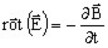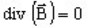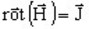E: electric field strength (in V/m)B: magnetic flux density (in T) H: magnetic field strength (in A/m) J: current density (in A/m2)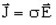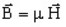σ : conductivity (in S) μ : permeability (in H/m)

The main equation for magnetic materials, can be put in form B(H) or H(B) as presented below.⇒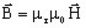μr : relative permeability μ0 : vacuum permeability
or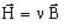⇒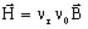νr : reluctivity νr =1/μr ν0 : vacuum reluctivity ν0 =1/μ0

## Models

To solve these equations, two models are used:

• the vector model, which uses: the magnetic vector potential (written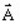)

• the scalar model, which uses: magnetic scalar potentials (written ϕtot or ϕred)

## Model and 2D or 3D application

The two models (vector and scalar) are proposed:
• the vector model for the 2D applications
• the scalar model for the 3D applications